AP State Syllabus AP Board 8th Class Maths Solutions Chapter 5 Comparing Quantities Using Proportion Ex 5.1 Textbook Questions and Answers.

## AP State Syllabus 8th Class Maths Solutions 5th Lesson Comparing Quantities Using Proportion Exercise 5.1Question 1.
Find the ratio of the following
(i) Smita works in office for 6 hours and Kajal works for 8 hours in her office. Find the
ratio of their working hours.
Solution:
The ratio of working hours of smita and kajal = 6:8
= (2 × 3 ) : (2 × 4) = 3 : 4

(ii) One pot contains 8 litre of milk while other contains 750 milliliter.
Solution:
8lit : 750ml
8 × 1000 : 750
= $$$\frac { 8000 }{ 750 }$$$ = 32 : 3

(iii) speed of a cycle is 15km/h and speed of the scooter is 30km/h.
Solution:
The ratio of speeds of a cycle and a sector
= 15 : 30 = (15 × 1) : 15 × 2 = 1 : 2Question 2.
If the compound ratio of 5:8 and 3:7 is 45:x. Find the value of x.
Solution:
The compound ratio of 5:8 and 3:7
= $$\frac{5}{8} \times \frac{3}{7}=\frac{15}{56}$$
According to the sum
15 : 56 = 45 : x
∴ x = 168

Question 3.
If the compound ratio of 7:5 and 8:x is 84:60. Find x.
Solution:
The compound ratio of 7:5 and 8:x
= $$\frac{7}{5} \times \frac{8}{x}=\frac{56}{5 x}$$
According to the sum
56 : 5x = 84 : 60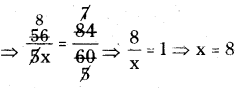∴ x = 8

Question 4.
The compound ratio of 3:4 and the inverse ratio of 4:5 is 45:x. Find x.
Solution:
The inverse ratio of 4:5 is 45 : x
The compound ratio of 3:4 and 5 : 4
= 45 : x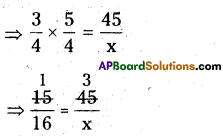⇒ x = 16 × 3 = 48
∴ x = 48Question 5.
In a primary school there shall be 3 teachers to 60 students. If there are 400 students
enrolled in the school, how many teachers should be there in the school in the same ratio?
Solution:
No. of teachers are required for 400 students at the rate of 3 teachers to 60
students are ⇒ 60 : 3 400 : x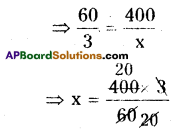∴ x = 20

Question 6.
In the given figure, ABC is a triangle. Write all possible ratios by A
taking measures of sides pair wise.
8cm 10cm
(Hint: Ratio of AB : BC =8 : 6)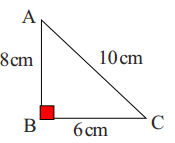Solution: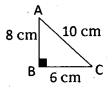In ΔABC
AB : BC = 8 : 6 = 4:3
⇒ BC : AB = 6 : 8 = 3: 4
BC : CA = 6 : 10 = 3 : 5
⇒ CA : BC = 10 : 6 = 5 : 3
CA : AB = 10:8=5:4
⇒ AB : CA = 8: 10 = 4: 5Question 7.
If 9 out of 24 students scored below 75% marks in a test. Find the ratio of student scored below 75% marks to the student scored 75% and above marks.
Solution:
Out of 24 students who got below 75% of marks = 9
Who got 75% and above marks =24 – 9 = 15
∴ The ratio between no. of students
who got less than 75% of marks and
who got 75% and above marks
= 9 : 15 =(3 × 3):(3 × 5) = 3 : 5

Question 8.
Find the ratio of number of vowels in the word’ MISSISSIPPI’ to the number of consonants in the simplest form.
Solution:
No. of vowels in the word MI S S SS! PPI = 4 (IIII)
No. of consonants in that word = 7 (MSSSSPP)
∴ The ratio between vowels and consonants = 4: 7

Question 9.
Rajendra and Rehana own a business. Rehana receives 25% of the profit in each month. If
Rehana received ₹ 2080 in particular month, what is the total profit in that month?
Solution:
Total Profit = x say
25% of x = 2080
⇒ $$\frac{25}{100}$$ × x = 2080
⇒ $$\frac{x}{4}$$ = 2080
⇒ x = 2080 × 4
∴ x = ₹ 8320Question 10.
In triangle ABC, AB = 2.2 cm, BC = 1.5 cm and AC = 2.3 cm. In triangle XYZ, XY = 4.4cm, YZ = 3cm and XZ = 4.6cm. Find the ratio AB:XY, BC:YZ, AC:XZ. Are the lengths of corresponding sides of ΔABC and ΔXYZ are in proportion?
[Hint : Any two triangles are said to be in proportion, if their corresponding sides are in the
same ratio]
Solution: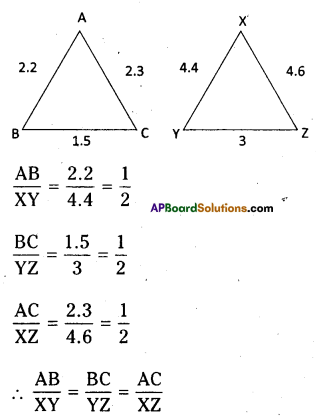∴ The corresponding sides of both the triangles are in proportion.
∴ ΔABC ~ ΔXYZ

Question 11.
Madhuri went to a super market. The price changes are as follows. The price of rice reduced by 5% jam and fruits reduced by 8% and oil and dal increased by 10%. Help Madhuri to find the changed prices in the given table.

 Item Original price/kg Changed price Rice ₹ 30 Jam ₹ 100 Apples ₹ 280 Oil ₹ 120 Dal ₹ 80

Solution:

 Item Original price/kg Changed price Rice ₹ 30 ₹28.50 Jam ₹ 100 ₹ 92 Apples ₹ 280 ₹ 257.6 Oil ₹ 120 ₹ 132 Dal ₹ 80 ₹ 88Question 12.
There were 2075 members enrolled in the club during last year. This year enrolment is
decreased by 4%.
(a) Find the decrease in enrolment.
(b) How many members are enrolled during this year?
Solution:
No. of persons are enrolled in the last year = 2075
Present year no. of persons are enrolled
= 4% less than the previous year.
a) Decrease in enrolment = 4% of 2075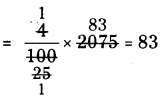b) No.of members are enrolled this
year = 2075 – 4% of 2075
=2075 – 83 = 1992

Question 13.
A farmer obtained a yielding of 1720 bags of cotton last year. This year she expects her crop to be 20% more. How many bags of cotton does she expect this year?
Solution:
During the last year yielding the bags of
cotton = 1720
If she expects 20% crop to be more then
=20% of 1720 .
= $$\frac{20}{100}$$ × 1720
= 2 × 172
= 344 bags
Her expectation of total bags
= 1720 + 344
= 2064Question 14.
Points P and Q are both in the line segment AB and on the same side of its midpoint. P divides AB in the ratio 2: 3, and Q divides AB in the ratio 3 :4. If PQ =2, then find the length of the line segment AB.
Solution:
Given that ‘C’ is the midpoint of line segment AB.
Here ‘P’ divides AB inthe ratio 2 : 3
‘Q’ divides AB in the ratio 3: 4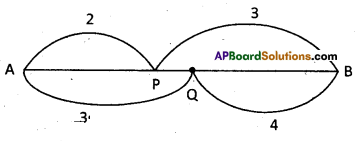PQ =2 cm [Given]
PQ = QB – PB
= 4 – 3 = 1 part = 2cm
∴ AB = AQ + QB [with respect to Ql
AB = AP+ PB [with respect to P]
L.C.M. of 5, 7 parts = 35 parts
∴ Length of AB 35 parts
= 35 × 2[ ∵ part = 2cm]
= 70cm